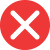33rd

Correct spelling, explanation: this form is the correct one because th is not the ending for ordinal numbers with 3 at the end. Since third ends with rd, this is the correct suffix for such numbers. Therefore 33rd is the correct form and 33th is incorrect.

Definition of 33rd:
numeral, the ordinal number between 32nd and 34th
-Which birthday do you have this year? –33rd.
He started to collect old cassettes last year; this one is his 33rd one.33th

Incorrect spelling, explanation: this form, 33th, is the wrong one, because we do not use this ending for ordinal numbers with third at the end. Th is the ending for numbers with th sound at the end, therefore 33rd is the correct form here. 33rd is correct because in the full-word form it is thirty-third.33nd

Incorrect spelling33st

Incorrect spelling